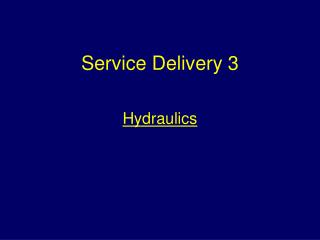Download PresentationService Delivery 3

# Service Delivery 3 - PowerPoint PPT PresentationDownload Presentation## Service Delivery 3

- - - - - - - - - - - - - - - - - - - - - - - - - - - E N D - - - - - - - - - - - - - - - - - - - - - - - - - - -
##### Presentation Transcript

1. Service Delivery 3 Hydraulics

2. Aim To ensure students can explain the principles of obtaining and delivering water.

3. Learning OutcomesAt the end of the session students will be able to: • Understand the relationship between atmospheric pressure and suction lift • Describe the principal characteristics of pressure • Describe how friction causes pressure loss • Explain the term ‘jet reaction’ • Carry out basic fireground calculations.

4. Area = wXh Area = vertical hXw a Area = a+bXh 2 b Calculating areas. w w h h

5. r h 2 Volume =   r  h Calculating volumes.

6. d h w Volume = hXwXd Calculating volumes.

7. Calculating volumes. Water Volume = 2 x a x d 3

8. Atmospheric Pressure Pressure is perpendicular to any surface on which it acts.

9. Pressure Gauges Pressure at any point in a fluid at rest is of the same intensity in all directions.

10. Pressure gauges Pressure gauges Pressure Pressure applied from outside to a fluid contained in a vessel is transmitted equally in all directions.

11. 3m 2m 1m Downward pressure of a fluid in an open vessel is proportional to depth.

12. The downward pressure of a fluid in an open vessel is proportional to the density of the fluid. Water 136mm Mercury 10mm

13. The downward pressure of a fluid on the bottom of a vessel is independent of the shape of the vessel.

14. vacuum Atmospheric pressure approx 1bar Water Lifting water by atmospheric pressure.

15. Theoretical lift Approximately 10 metres - assuming a perfect vacuum can be created and the water is cool and pure.

16. Pressure and head • The height to which water is lifted or pumped is referred to as the head • To raise a column of water 1 metre in height requires a pressure of 0.1bar. This applies to both ‘suction head’ and ‘delivery head’.

17. Factors limiting suction lift • Lifting water from it’s existing level to the pump inlet • Overcoming frictional resistance to the water • Turbulence at the pump eye • Creation of flow.

18. Practical lift Because of these factors the maximum practical suction lift is generally regarded as 8 metres.

19. 7m 2m 8m 3m water water water Lifting practically.

20. 0.4b Friction loss 0.2b 1 2 Hose lengths Friction loss varies directly with the length of the hose .

21. 45mm 70mm 90mm. For the same velocity friction loss decreases with the increase in diameter

22. Friction loss increases directly with the square of the velocity 2000 litres/min pressure loss 6bar 1000 litres/min pressure loss 1.5bar.

23. Calculating jet pressures Pressure loss due to friction; • 70mm non-percolating hose 0.2 bars per 25 metre length.

24. Pressure loss due to head For every metre in height that water is pumped 0.1bar of pressure is lossed.

25. Question 6 lengths of hose are being used to supply a branch deployed at a height of 15m What pressure will be required at the pump to supply 3 bars pressure at the branch.

26. Answer Nozzle pressure required 6 bars Pressure loss due to head 0.1b X 15 = 1.5b Pressure loss due to friction 0.2b X 6 = 1.2b Pump pressure required 8.7bars

27. Fireground flow rate calculation • 45mm diameter hose = 300 litres/min • 70mm diameter hose = 600 litres/min

28. Function of a branch and nozzle To convert pressure energy into velocity energy and ensure that an effective jet of water leaves the nozzle.

29. 45mm diameter hoseline 20mm diameter nozzle. Achieved by reducing the cross-sectional area that the water is flowing through

30. Advantages of higher nozzle pressures • A greater striking power is achieved • A longer reach can be obtained • A larger volume of water is applied.

31. Factors affecting the pressures adopted • The type and size of nozzle used • The type of jet required • The intensity of the wind.

32. Jet Reaction The magnitude of the reaction is dependant on; • The mass of water per second passing through the nozzle • The squared diameter of the nozzle.

33. Jet Reaction

34. Jet Reaction = 1.57 x p x d 10 2

35. R = 1.57× 7 × (25x25) 10 = 687 Newtons. 1.57 x p x d 10 2 Using a 25mm nozzle at 7 bars pressure

36. R = 1.57× 7 × (12.5x12.5) 10 = 172 Newtons. 1.57 x p x d 10 2 Using a 12.5mm nozzle at 7 bars pressure

37. ConfirmationAssessments will be based on this lesson and the corresponding study note Learning Outcomes • Understand the relationship between atmospheric pressure and suction lift • Describe the principal characteristics of pressure • Describe how friction causes pressure loss • Explain the term ‘jet reaction’ • Carry out basic fireground calculations.

38. THE END